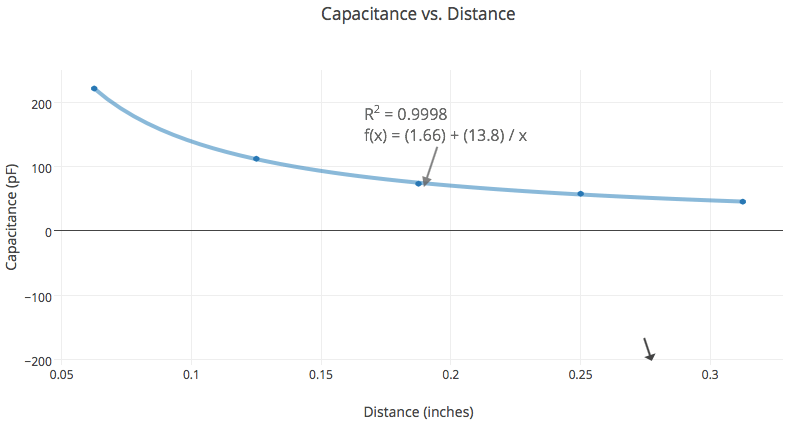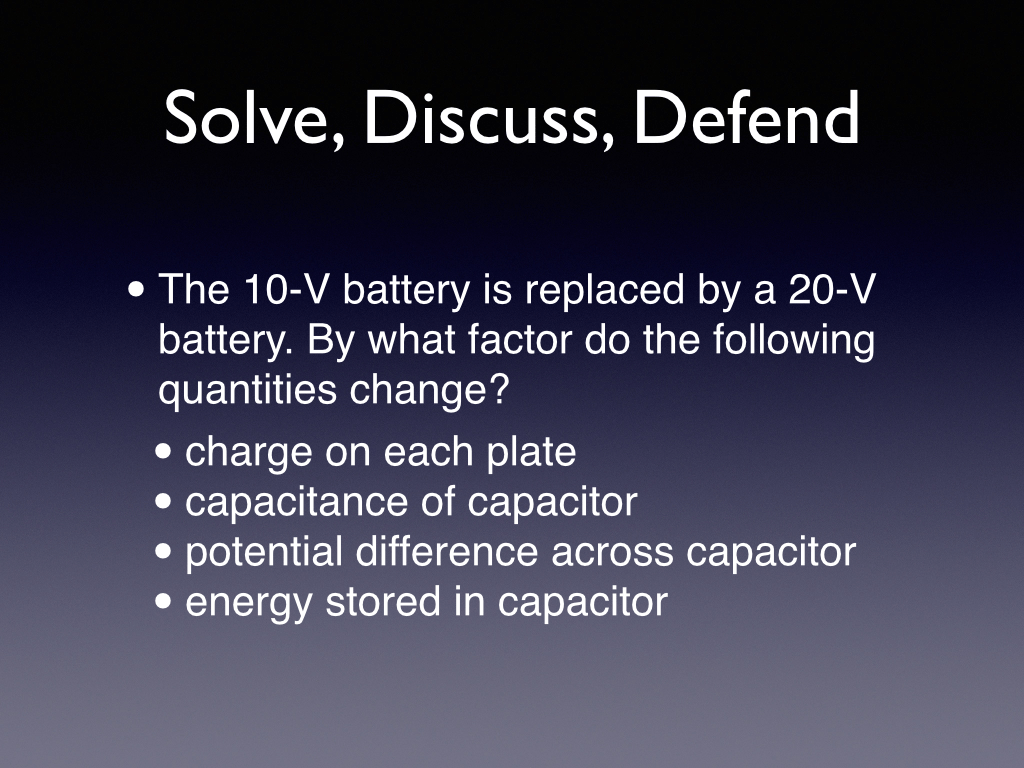# Day 56: Capacitance

Today in AP Physics 2, we discussed the results of the capacitor paradigm lab. The graphs clearly showed an inverse relationship between capacitance and distance. The plot of capacitance vs. area was linear, but not as good a fit.After formally defining capacitance and deriving the various capacitance-related equations, I asked students a series of proportional reasoning questions:The most thought-provoking follow-up question was after the last slide when I asked if the stored energy in the capacitor doubled, where did the energy come from?